# What Is A Zener Diode And How It Is Used As A Voltage Stabiliser

## What Is A Zener Diode

When the reverse bias voltage on a normal crystal diode is increased, a point known as Knee point is reached at a  voltage known as breakdown voltage, when the  reverse current increases sharply to a high value. This breakdown voltage is also known as  Zener Voltage and the sharp increase in current is known as Zener current.

The breakdown voltage of a diode depends on the amount of doping. In a heavily doped diode, the depletion layer is thin, so the breakdown of the junction occurs at a lower reverse bias voltage. On the other hand, a lightly doped diode has a higher breakdown voltage. When an ordinary crystal diode is properly doped so as to have a sharp breakdown voltage ,it is known  as  zener diode. Fig(1) shows the symbol of a zener diode.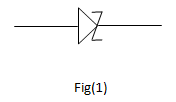A zener diode is always used in reverse biased state. It  has sharp breakdown voltage known as Zener voltage  VZ. When forward biased ,it behaves just like ordinary crystal diode. Fig(2)  shows the characteristics of a zener diode.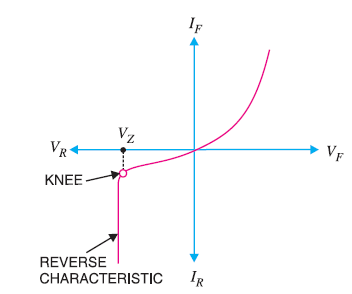Fig. 2

### Equivalent Circuit of  a Zener Diode

#### Case 1. On State

When the reverse voltage across a zener diode is equal to or higher than the breakdown voltage VZ , the current increase very sharply.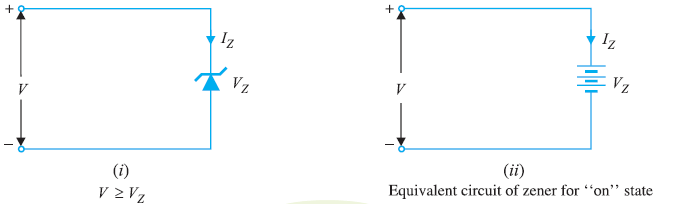Fig. 3

In this state, voltage across the zener diode is constant at VZ irrespective of the change in current though it.

Therefore, in breakdown region an ideal zener diode acts as a battery of voltage  VZ , and the zener diode is said to be in “ON” State.

#### Case 2.OFF  State:

When the reverse voltage across the zener diode is less than VZ but   greater than 0v, the zener diode is in the “OFF”State. Under such state, the zener diode can be replaced by an Open circuit.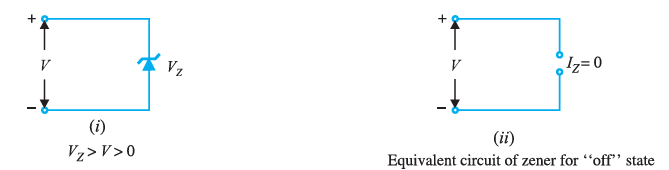Fig. 4

## Zener Diode as Voltage Stabiliser

A zener diode can be used as a voltage stabiliser or voltage regulator to provide a constant voltage from a source whose voltage may vary over a particular range.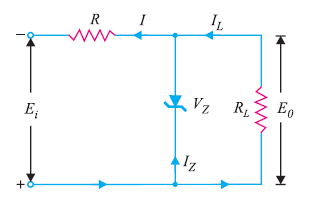Fig. 5

The circuit connection is shown in fig(5).

The zener diode of zener voltage  VZ is connected reversly across the load resistance  RL across which constant output voltage EO is required.

The series resistance R is used to absorb the output voltage fluctuations, so as to maintain constant output voltage across RL .

When the circuit is properly designed, the output voltage EO remains constant even though the input voltage  Ei and load resistance RL may vary over a wide range.

#### Case 1: Ei Variable  and RL constant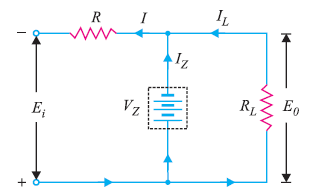Fig.6

Suppose the input voltage Ei increases, since the zener is in the breakdown region, the zener diode is equivalent to a battery of voltage VZ and the output voltage remains constant at VZ ( EO =VZ).

The excess voltage is dropped across R. This will cause an increase in the value of total current I. The zener will conduct the increase of current in I, while the load current remains constant.

Hence the output voltage remains constant irrespective of the change in input voltage Ei

#### Summary(Case 1)

Suppose  Ei  increases

Since zener is in breakdown region, VZ remains constant

EO = VZ

So  EO   remains constant

Excess voltage drops across R

So I increases, since   IL remains constant, IZ increases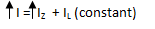So the excess current is conducted by the zener diode and EO remains constant irrespective of the change in Ei

#### Case 2: Ei Constant  and RL Vaiable

Now suppose the input voltage Ei is constant but RL decreases

Since the zener diode is in breakdown region , voltage across it will remain constant at   VZ.

As the output voltage EO is equal to the zener  voltage, So EO will also remain constant at VZ.

When RL  decreases ,in order  to maintain EO constant, current through the load resistance  IL  will increase.

Since Ei is constant, total current  I is also constant. So the increase in load current IL will come from a decrease in zener current IZ  .Voltage drop across R =   Ei   – EO

Current through R , I = IZ + IL